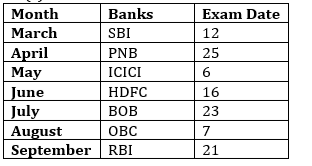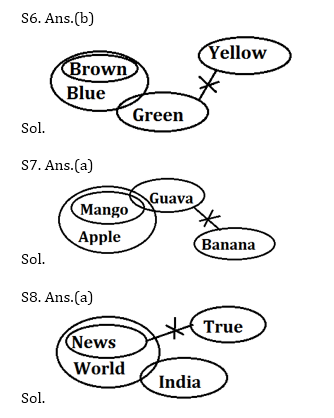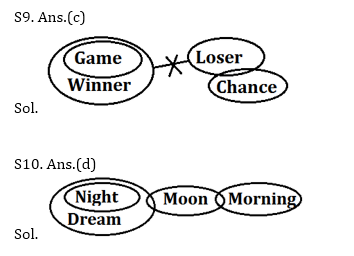Latest Banking jobs   »   Reasoning Ability Quiz For ECGC PO...

# Reasoning Ability Quiz For ECGC PO 2021- 16th January

Directions (1-5): Study the information carefully and answer the questions given below.
Seven banks conduct entrance exam on seven different dates of the seven different months starting from March to September of the same year.
SBI conducts exam on the date which is completely divisible by 3 and 4, and in the month, which has odd number of days. HDFC conducts exam in the month which has even number of days and on the date, which is square of the even number. More than two banks are conducting exam after ICICI bank. OBC conduct exam 15days after BOB’s exam and in the month, which have 31days. Three banks conduct their exam between PNB and OBC. ICICI does not conduct exam in March. Difference between the dates of HDFC and PNB is 9. PNB conducts exam before ICICI and on the date which is square of an odd number. The bank which conducts the exam on the 21st of the month, takes it immediately after the OBC Bank. Difference between the dates of SBI and RBI is 9. The bank which conducts exam in August conducts on 7th of the month. ICICI bank conducts exam on 6th of the month.

Q1. Difference between the exam date of RBI and OBC?
(a) 8
(b) 19
(c) 14
(d) 7
(e) None of these

Q2. SBI conducts exam in which of the following month?
(a) May
(b) March
(c) September
(d) August
(e) None of these

Q3. How many banks conducts exam before BOB bank?
(a) One
(b) Two
(c) Four
(d) Three
(e) None

Q4. Which of the following bank conducts exam in September?
(a) SBI
(b) HDFC
(c) BOB
(d) OBC
(e) None of these

Q5. Which of the following combination is true about PNB?
(a) June-23
(b) May-9
(c) July-7
(d) April-25
(e) None is true

Directions (6-10): In these questions some statements are followed by some conclusions numbered. You have to take the three given statements to be true even if they seem to be at variance from commonly known facts and then decide which of the given conclusions logically follows from the given statements disregarding commonly known facts.

Q6. Statements:
Only a few Blue are Green.
No Green is a Yellow.
Only Blue is Brown.
Conclusions:
I. Some Brown is Yellow is a possibility
II. Some Yellow is Blue is a possibility
(a) Only I follows.
(b) Only II follows.
(c) Both I and II follow.
(d) Either I or II follows.
(e) Neither I nor II follows.

Q7. Statements:
All Mango are Apple.
Only Few Guava are Mango.
No Guava is Banana
Conclusions:
I. All Banana is Mango is a possibility
II. Some Apple is Guava is a possibility
(a) Only I follows.
(b) Only II follows.
(c) Either I or II follows.
(d) Neither I nor II follows.
(e) Both I and II follow.

Q8. Statements:
All News is World.
Some World is India.
No News is True.
Conclusions:
I. All True is India
II. No True is India
(a) Either I or II follows.
(b) Both I and II follow.
(c) Only I follows.
(d) Neither I nor II follows.
(e) Only II follows.

Q9. Statements:
All Game are Winner.
No Winner are Loser.
Only a few Loser is Chance.
Conclusions:
I. All Loser is Chance
II. Atleast some Winner is Chance
(a) Either I or II follows.
(b) Only II follows.
(c) Neither I nor II follows.
(d) Only I follows.
(e) Both I and II follow.

Q10. Statements:
Only Dream is Night.
Only a few Moon is Dream.
Only a few Moon is Morning.
Conclusions:
I. All Morning is Moon is a possibility
II. All Moon is Dream is a possibility
(a) Either I or II follows.
(b) Both I and II follow.
(c) Neither I nor II follows.
(d) Only I follow.
(e) Only II follows.

Directions (11-15): Study the following information carefully and answer the given questions.
In number arrangement machine when given an input line of numbers, it rearranges them following a particular rule in each step. The following is an illustration of input and rearrangement.

Input: 12 75 28 36 93 44 51
Step I: 13 72 29 37 90 45 48
Step II: 90 72 48 45 37 29 13
Step III: 9 5 4 1 4 7 2
Step IV: 18 10 12 2 12 14 6

And step IV is the last step of the arrangement.
As per the above rule followed in the above steps, find out in each of the following
questions the appropriate step for the input given below;

Input: 86 17 57 26 65 43 72

Q11. Which of the following digit is fifth from the right end in step-III?
(a) 4
(b) 1
(c) 5
(d) 3
(e) None of these

Q12. What is the sum of the numbers which are second from the left end in step-II and third to the right end in step IV?
(a) 21
(b) 85
(c) 87
(d) 56
(e) None of these

Q13. How many prime numbers are obtained in last step?
(a) Four
(b) One
(c) Two
(d) Three
(e) None

Q14. What is the multiplication of the digits of the number which is third from the right end in step II?
(a) 18
(b) 10
(c) 21
(d) 25
(e) None of these

Q15. What is the difference between the numbers which is third from the left end in last step and second from the right end in first step?
(a) 7
(b) 27
(c) 31
(d) 40
(e) None of these

Practice More Questions of Reasoning for Competitive Exams:

###### ECGC PO Study Plan 2021

Solutions

Sol. (1-5)S1. Ans.(c)
S2. Ans.(b)

S3. Ans.(c)

S4. Ans.(e)

S5. Ans.(d)S11. Ans.(a)
Sol.
Let us understand the logic behind it-
* In Step-1, one is added in each even digit and three is subtracted in each odd digit of the input.
* In Step-2, All the numbers of step-1 arranged in descending order from left to right.
* In Step-3, Digits of the number are subtracted with in the number which are obtained in step-2.
* In Step-4, two is multiply by each odd digit and three is multiply by each even digit which are obtained in step-3.

Input: 86 17 57 26 65 43 72
Step I: 87 14 54 27 62 40 73
Step II: 87 73 62 54 40 27 14
Step III: 1 4 4 1 4 5 3
Step IV: 2 12 12 2 12 10 6

S12. Ans.(b)
Sol.
Let us understand the logic behind it-
* In Step-1, one is added in each even digit and three is subtracted in each odd digit of the input.
* In Step-2, All the numbers of step-1 arranged in descending order from left to right.
* In Step-3, Digits of the number are subtracted with in the number which are obtained in step-2.
* In Step-4, two is multiply by each odd digit and three is multiply by each even digit which are obtained in step-3.

Input: 86 17 57 26 65 43 72
Step I: 87 14 54 27 62 40 73
Step II: 87 73 62 54 40 27 14
Step III: 1 4 4 1 4 5 3
Step IV: 2 12 12 2 12 10 6

S13. Ans.(c)
Sol.
Let us understand the logic behind it-
* In Step-1, one is added in each even digit and three is subtracted in each odd digit of the input.
* In Step-2, All the numbers of step-1 arranged in descending order from left to right.
* In Step-3, Digits of the number are subtracted with in the number which are obtained in step-2.
* In Step-4, two is multiply by each odd digit and three is multiply by each even digit which are obtained in step-3.

Input: 86 17 57 26 65 43 72
Step I: 87 14 54 27 62 40 73
Step II: 87 73 62 54 40 27 14
Step III: 1 4 4 1 4 5 3
Step IV: 2 12 12 2 12 10 6

S14. Ans.(a)
Sol.
Let us understand the logic behind it-
* In Step-1, one is added in each even digit and three is subtracted in each odd digit of the input.
* In Step-2, All the numbers of step-1 arranged in descending order from left to right.
* In Step-3, Digits of the number are subtracted with in the number which are obtained in step-2.
* In Step-4, two is multiply by each odd digit and three is multiply by each even digit which are obtained in step-3.

Input: 86 17 57 26 65 43 72
Step I: 87 14 54 27 62 40 73
Step II: 87 73 62 54 40 27 14
Step III: 1 4 4 1 4 5 3
Step IV: 2 12 12 2 12 10 6

S15. Ans.(e)
Sol.
Let us understand the logic behind it-
* In Step-1, one is added in each even digit and three is subtracted in each odd digit of the input.
* In Step-2, All the numbers of step-1 arranged in descending order from left to right.
* In Step-3, Digits of the number are subtracted with in the number which are obtained in step-2.
* In Step-4, two is multiply by each odd digit and three is multiply by each even digit which are obtained in step-3.

Input: 86 17 57 26 65 43 72
Step I: 87 14 54 27 62 40 73
Step II: 87 73 62 54 40 27 14
Step III: 1 4 4 1 4 5 3
Step IV: 2 12 12 2 12 10 6

Practice with Crash Course and Online Test Series for ECGC PO 2021:×

Thank You, Your details have been submitted we will get back to you.Join India's largest learning destination

What You Will get ?

•Job Alerts
•Daily Quizzes
•Subject-Wise Quizzes
•Current Affairs
•Previous year question papers
•Doubt Solving session

ORJoin India's largest learning destination

What You Will get ?

•Job Alerts
•Daily Quizzes
•Subject-Wise Quizzes
•Current Affairs
•Previous year question papers
•Doubt Solving session

ORJoin India's largest learning destination

What You Will get ?

•Job Alerts
•Daily Quizzes
•Subject-Wise Quizzes
•Current Affairs
•Previous year question papers
•Doubt Solving session

Enter the email address associated with your account, and we'll email you an OTP to verify it's you.Join India's largest learning destination

What You Will get ?

•Job Alerts
•Daily Quizzes
•Subject-Wise Quizzes
•Current Affairs
•Previous year question papers
•Doubt Solving session

Enter OTP

Please enter the OTP sent to
/6

Did not recive OTP?

Resend in 60sJoin India's largest learning destination

What You Will get ?

•Job Alerts
•Daily Quizzes
•Subject-Wise Quizzes
•Current Affairs
•Previous year question papers
•Doubt Solving sessionJoin India's largest learning destination

What You Will get ?

•Job Alerts
•Daily Quizzes
•Subject-Wise Quizzes
•Current Affairs
•Previous year question papers
•Doubt Solving session

Almost there

+91Join India's largest learning destination

What You Will get ?

•Job Alerts
•Daily Quizzes
•Subject-Wise Quizzes
•Current Affairs
•Previous year question papers
•Doubt Solving session

Enter OTP

Please enter the OTP sent to Edit Number

Did not recive OTP?

Resend 60

By skipping this step you will not recieve any free content avalaible on adda247, also you will miss onto notification and job alerts

Are you sure you want to skip this step?

By skipping this step you will not recieve any free content avalaible on adda247, also you will miss onto notification and job alerts

Are you sure you want to skip this step?